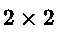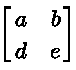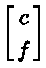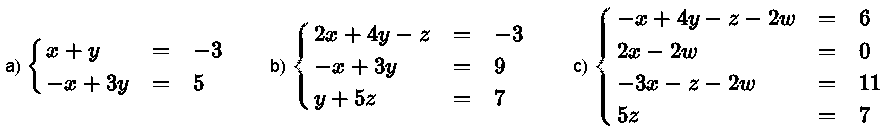# The Three Row Operations on Augmented Matrices

## What is an Augmented Matrix?

The augmented matrix of a system of linear equations is made up of the matrix of the coefficients of the unknowns augmented by the matrix of the constants .
Let asystem of linear equations, with the unknowns, be given byWrite the above system of linear equations using matrices.The augmented matrix of the above system is given byNote the vertical separators between the matrix of the coefficient and the matrix of the constants.is the matrix of the coefficients of the variables andis the matrix of the constants

Example 1
Write the augmented matrix for the given systems.Solution to Example 1
The augmented matrices of the given systems of equations are:Note that all the information in the system of equations is included in the augmented matrix as coefficients and constants and therefore we can solve a given system of linear equations using its augmented matrix. The advantage of using augmented matrices instead of systems of equations is that augmented matrices can be manipulated using computers without worrying about the variables.

Example 2  Write the systems of equations whose augmented matrices are given below.
a) $$\begin{bmatrix} 0 & 1 &|& 4\\ -2 & 0 &|& 0 \end{bmatrix}$$     b) $$\begin{bmatrix} -9 & 0 & 0 &|& 4\\ 0 & 3 & 0 &|& -1 \\ 1 & -3 & 9 &|& -7 \end{bmatrix}$$     c) $$\begin{bmatrix} -4 & 0 & -1 & 0 &|& -4\\ 7 & 0 & -2 & 7 &|& -2\\ 4 & 0 & 0 & -8 &|& 0 \\ -1 & -5 & 7 & 0 &|& -1 \end{bmatrix}$$

Solution to Example 2
a) $$\left\{ \begin{array}{lcl} y & = & 4 \\ -2x & = & 0 \end{array} \right.$$     b) $$\left\{ \begin{array}{lcl} -9x & = & 4 \\ 3y & = & -1 \\ x -3y + 9z & = & -7 \end{array} \right.$$     c) $$\left\{ \begin{array}{lcl} -4x - z & = & -4 \\ 7x - 2 z + 7 w & = & -2 \\ 4x - 8w & = & 0 \\ -x -5 y + 7z & = & -1 \end{array} \right.$$

## The Three Row Operations on Augmented Matrices

We now show that the three elementary operations on the equations of a system have three equivalent elementary operations on the rows of the augmented matrix of the system.

Operations on Equations Operations on Rows of Augmented Matrix
1. Interchange two equations
2. Add a multiple of one equation to another
3. Multiply an equation by a non zero constant
1. Interchange two rows
2. Add a multiple of one row to another
3. Multiply a row by a non zero constant

An example that shows that the two types of operations are equivalent is presented below.
Example 3
Use operations on equations and the same operations on rows of the augmented matrix of the system of linear equations given below and show that the systems obtained correspond to the augmented matrices obtained.
$$\left\{ \begin{array}{lcl} x + y - z & = & -3 \\ -x + 3y + 2z & = & 5 \\ x + 5z & = & 9 \end{array} \right.$$

Solution to Example 3
In what follows, equations in the system and rows in the augmented matrix are ranked as follows: equation (1) is the top equation, equation (2) is the second equation from the top and so on and similarly for the rows; row (1) is the top row, row (2) is the second row from the top and so on.

 System of Equations Corresponding Augmented Matrix Given: $$\left\{ \begin{array}{lcl} x + y - z & = & -3 \\ -x + 3y + 2z & = & 5 \\ x + 5z & = & 9 \end{array} \right.$$ $$\begin{bmatrix} 1 & 1 & - 1 &|& -3\\ -1 & 3 & 2 &|& 5 \\ 1 & 0 & 5 &|& 9 \end{bmatrix}$$ add equation (1) to equation (2) Add row (1) to row (2) $$\left\{ \begin{array}{lcl} x + y - z & = & -3 \\ 0x + 4y + z & = & 2 \\ x + 5z & = & 9 \end{array} \right.$$ $$\color{red}{\begin{matrix} \\ R_2 + R_1\\ \\ \end{matrix}}$$ $$\begin{bmatrix} 1 & 1 & - 1 &|& -3\\ 0 & 4 & 1 &|& 2 \\ 1 & 0 & 5 &|& 9 \end{bmatrix}$$ add $$-1$$ times equation (1) to equation (3) Add $$-1$$ times row (1) to row (3) $$\left\{ \begin{array}{lcl} x + y - z & = & -3 \\ 0x + 4y + z & = & 2 \\ 0x - y + 6 z & = & 12 \end{array} \right.$$ $$\color{red}{\begin{matrix} \\ \\ R_3 - R_1\\ \end{matrix}}$$ $$\begin{bmatrix} 1 & 1 & - 1 &|& -3\\ 0 & 4 & 1 &|& 2 \\ 0 & -1 & 6 &|& 12 \end{bmatrix}$$ Interchange equations (2) and (3) Interchange rows (2) and (3) $$\left\{ \begin{array}{lcl} x + y - z & = & -3 \\ 0x - y + 6 z & = & 12\\ 0x + 4y + z & = & 2 \end{array} \right.$$ $$\color{red}{\begin{matrix} \\ R_3\\ R_2\\ \end{matrix}}$$ $$\begin{bmatrix} 1 & 1 & - 1 &|& -3\\ 0 & -1 & 6 &|& 12\\ 0 & 4 & 1 &|& 2 \end{bmatrix}$$ Add 4 times equation (2) to equation (3) Add 4 times row (2) to row (3) $$\left\{ \begin{array}{lcl} x + y - z & = & -3 \\ 0x - y + 6 z & = & 12\\ 0x + 0y + 25z & = & 50 \end{array} \right.$$ $$\color{red}{\begin{matrix} \\ \\ R_3 + 4R_2\\ \end{matrix}}$$ $$\begin{bmatrix} 1 & 1 & - 1 &|& -3\\ 0 & -1 & 6 &|& 12\\ 0 & 0 & 25 &|& 50 \end{bmatrix}$$ Multiply all terms in equation (2) by $$-1$$ Multiply all terms in row (2) by $$-1$$ $$\left\{ \begin{array}{lcl} x + y - z & = & -3 \\ 0x + y - 6 z & = & - 12\\ 0x + 0y + 25z & = & 50 \end{array} \right.$$ $$\color{red}{\begin{matrix} \\ -R_2\\ \\ \end{matrix}}$$ $$\begin{bmatrix} 1 & 1 & - 1 &|& -3\\ 0 & 1 & - 6 &|& - 12\\ 0 & 0 & 25 &|& 50 \end{bmatrix}$$ Multiply all terms in equation (3) by $$\dfrac{1}{25}$$ Multiply all terms in row (3) by $$\dfrac{1}{25}$$ $$\left\{ \begin{array}{lcl} x + y - z & = & -3 \\ 0x + y - 6 z & = & - 12\\ 0x + 0y + z & = & 2 \end{array} \right.$$ $$\color{red}{\begin{matrix} \\ \\ (1/25)R_3\\ \end{matrix}}$$ $$\begin{bmatrix} 1 & 1 & - 1 &|& -3\\ 0 & 1 & - 6 &|& - 12\\ 0 & 0 & 1 &|& 2 \end{bmatrix}$$

All the augmented matrices obtained on the right correspond to the system of equations on the left and we can therefore solve any system of linear equations using augmented matrices.
Rows operations are used to rewrite augmented matrices in reduced row echelon form which is itself used to solve systems of equations, find inverse of matrices, check the linearity of vectors in linear algebra, find the change of basis matrices, find null, column and row spaces of matrices.

## Questions with Solution

• Part 1
Find the augmented matrix for each of the following systems of linear equations.
1. $$\left\{ \begin{array}{lcl} x + 2 y & = & 2 \\ - 2x + 4y & = & -1 \end{array} \right.$$

2. $$\left\{ \begin{array}{lcl} - x + 2 y + z & = & -5 \\ - x - \dfrac{1}{3}y & = & - 7\\ -\dfrac{2}{5} y - 9 z & = & 0 \end{array} \right.$$

3. $$\left\{ \begin{array}{lcl} 2 x + z - w & = & 0\\ - \dfrac{1}{2} y - 4z & = & -4\\ x - 7y & = & 7 \\ - 4y - 5z -\dfrac{1}{5} w& = & 2 \end{array} \right.$$

• Part 2
Find the system of linear equations given the augmented matrix.
1. $$\begin{bmatrix} 1 & -2 & |& -8\\ 9 & -1 &|& 0 \end{bmatrix}$$

2. $$\begin{bmatrix} -1 & 1 & - 1 &|& -6\\ 1& 0 & -2 &|& 4 \\ 0 & -1 & 7 &|& -4 \end{bmatrix}$$

3. $$\begin{bmatrix} -\dfrac{2}{3} & -1 & 1 & - 8 &|& 2\\ 0 & -\dfrac{1}{2} & -4 & \dfrac{1}{3} &|& 7\\ -1 & 3 & 0 & 0 &|& 7 \\ 0 & - 5 & 8 & \dfrac{4}{5} &|& 2 \end{bmatrix}$$

Solutions to the Above Questions

• Part 1
1. $$\begin{bmatrix} 1 & 2 &|& 2\\ -2 & 4 &|& - 1 \end{bmatrix}$$

2. $$\begin{bmatrix} -1 & 2 & 1 &|& -5\\ -1& -\dfrac{1}{3} & 0 &|& - 7\\ 0 & -\dfrac{2}{5} & -9 &|& 0 \end{bmatrix}$$

3. $$\begin{bmatrix} 2 & 0 & 1 & -1 &|& 0\\ 0 & -\dfrac{1}{2} & -4 & 0 &|& - 4\\ 1 & -7 & 0 & 0 &|& 7 \\ 0 & -4 & -5 & -\dfrac{1}{5} &|& 2 \end{bmatrix}$$

• Part 2
1. $$\left\{ \begin{array}{lcl} x - 2 y & = & -8 \\ 9x - y & = & 0 \end{array} \right.$$

2. $$\left\{ \begin{array}{lcl} - x + y - z & = & -6 \\ x - 2z & = & 4\\ - y +7z & = & -4 \end{array} \right.$$

3. $$\left\{ \begin{array}{lcl} -\dfrac{2}{3} x - y + z - 8 w & = & 2\\ - \dfrac{1}{2} y - 4z + \dfrac{1}{3} w& = & 7\\ - x + 3y & = & 7 \\ - 5y + 8z + \dfrac{4}{5} w& = & 2 \end{array} \right.$$